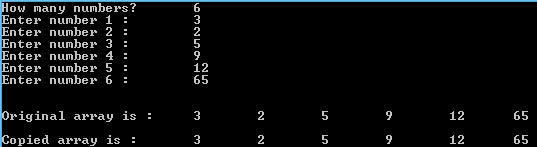## Friday, 9 January 2015

### C Program to Copy the elements of one array to another

This is a C Program which copies elements from one array and place it another array.

Here the elements of the first array are read one by one and copied into another array , and then prints the copied array.

PROGRAM :
```#include <stdio.h>
#include <stdlib.h>

int main()
{
int i,n;
printf("How many numbers? \t");
scanf("%d",&n);
int a[n],b[n];
for(i=0;i<n;i++)
{
printf("Enter number %d : \t",i+1);
scanf("%d",&a[i]);
}
printf("\n\nOriginal array is :\t");
for(i=0;i<n;i++)
printf("%d \t",a[i]);

for (i = 0; i < n; i++)
b[i] = a[i];

printf("\n\nCopied array is :\t");
for(i=0;i<n;i++)
printf("%d \t",b[i]);

return 0;
}```

OUTPUT :C - Coping elements from one array to another